Courses

# Creep and Shrinkage Deformation Civil Engineering (CE) Notes | EduRev

## Civil Engineering (CE) : Creep and Shrinkage Deformation Civil Engineering (CE) Notes | EduRev

The document Creep and Shrinkage Deformation Civil Engineering (CE) Notes | EduRev is a part of Civil Engineering (CE) category.
All you need of Civil Engineering (CE) at this link: Civil Engineering (CE)

Creep and Shrinkage Deformation
→ Creep of concrete
o Concrete under stress undergoes a gradual increase of strain with time. The final creep strain may be several times as large as the initial elastic strain.
o Creep is the property of materials by which they continue deforming over considerable length of time under sustained stress.
o Relaxation is the loss of stress by time with constant strain.
o In concrete, creep deformations are generally larger than elastic deformation and thus creep represents an important factor affecting the deformation behavior.
o Concrete under constant axial compressive stress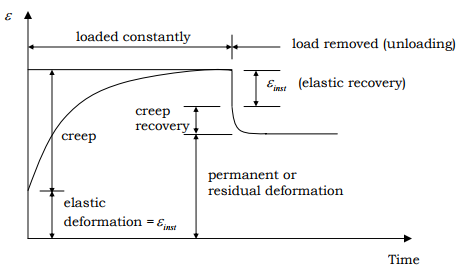→ Experiments indicate that for working stress range – i.e. stresses not exceeding 0.5 f'c– creep strains are directly proportional to stress that is a linear relationship between σ and εcr .
→ Microcracking effect on creep in high stresses.

→ Mechanisms of concrete creep
o Two phenomena are involved:
1. Time dependent deformation that occurs when concrete is loaded in a sealed condition so that moisture cannot escape. → basic creep
2. Creep of the material when moisture exchange is permitted. → drying creep

o Basic creep is primarily influenced by the material properties only, while drying creep and shrinkage also depend on the environment and the size of the specimen.
o The real situation might be the combination of the two phenomena, sometimes, one being the dominating factor.
o Creep deformation contains three regions:
1. Primary creep → initial increase in deformation
2. Secondary creep → relatively a steady deformation region
3. Tertiary creep → leads to creep fracture

→ Specific creep
o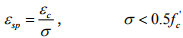where ε= a function of time. → Stress levels above 0.8f'c creep produces failure in time.
o Relationship between f'c and εsp :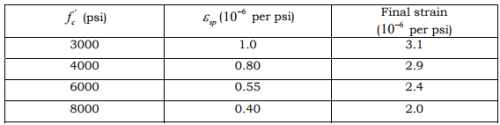→ Creep coefficient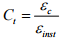where εinst = instantaneous (initial) strain.

Factors influencing creep:
o Internal factors (composition)
→ Aggregate (concentration + stiffness)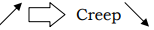→ Water/cement ratio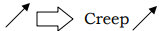→ Aggregate permeability→ Aggregate creep Creep→ Aggregate stiffness Creep→ Aggregate grading and distribution
→ Cement
o External factors (environment, time history)
→ Size
→ Shape
→ Cross-section→ Environmental factors (ambient humidity, temperature)
→ Stress intensity→ Time (age of loading) → the loading history is important to the total deformation (strain).
→ age of loading Creep→ Mathematical modeling of creep:
o Strain decomposition
The total strain of concrete may be decomposed as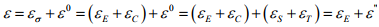where
εσ = stress-induced strain,
εE = reversible strain,
εC = creep strain,
ε​0 = stress-independent inelastic strain,
εS = shrinkage strain,
εT = thermal expansion, and
ε" = inelastic strain.

o Incremental formulation of stress-strain relation
{dσ} = [D]{dε}

where {dσ} = change in stress tensor,
[D] = constitutive law of the material, and
{dε} = change in strain tensor.

o First-order and higher orders formulations of creep and shrinkage deformation:
→ First-order formulations: The incremental elastic stiffness matrix changes from one time step to the next as proportional to the elastic modulus. Applicable to homogeneous materials.
→ Higher order formulations: The incremental elastic stiffness matrix is different and not proportional to the elastic modulus from one time step to another. Applicable to non-homogeneous materials.

o Incremental quasi-elastic stress-strain law

∆ε = J∆σ + ∆ε0

where
∆ε = a column matrix consisting of the strain increments,
J = compliance (square) matrix (function),
∆σ = a column matrix consisting of the stress increments, and
∆ε0 = a column matrix consisting of the inelastic strain increments.

→ Linear methods for the calculation of creep strain
o Effective modulus method
Total stress-strain relation: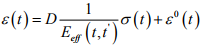where t = current time,
t' = the time at which the instantaneous elastic modulus is characterized,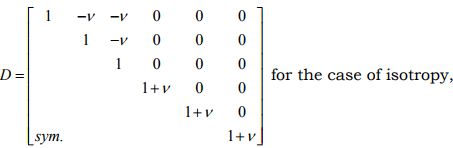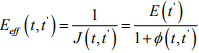= effective modulus of elasticity,
ε(t) = stress-independent inelastic strain,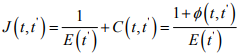compliance function (the creep function) representing the strain (elastic + creep) at time t

E (t')= modulus of elasticity characterizing the instantaneous deformation at time , t'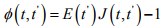= creep coefficient representing the ratio of the creep deformation to the initial elastic deformation, and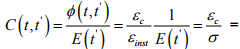specific creep function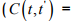εsp).

→ Incremental strain The method is applied incrementally from tto  t if the structural system is changed after .t0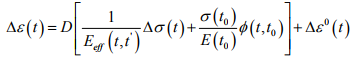where ∆σ(t) = incremental form of the stress at time t,
∆ε0(t) = incremental form of the stress-independent inelastic strain, and
t0 = time of first loading.

→ Effective modulus method is exact when the stress is constant from t' to t.

o Linear variation method
→ Assuming the mechanical strain ε −ε0 is zero up to time tand jumps to the value of a, apply linear variation of strain from tto  t and obtain the following expression: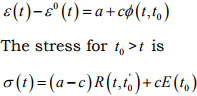where a, c = constants.

One can then obtain by algebraic manipulation the basic incremental stress-strain relation of the age-adjusted effective modulus.

o Age-adjusted effective modulus method
Effective elastic modulus with a correction of the creep coefficient: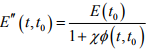where χ = the relaxation coefficient (aging coefficient, age-adjusted effective modulus) = 0.5~1.0.

The quasi-elastic incremental stress-strain relation of the ageadjusted effective modulus: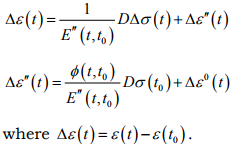We assume isotropic material with a constant Poisson’s ratio, which is approximately true for concrete.

→ Practical considerations of creep prediction
o Considering the specific strain function expressed as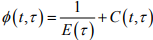That is strain per unit stress at time t for the stress applied at age τ .

o The specific creep function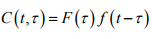o Forms of the aging function F (τ ) and f (t −τ ) :

→ F (τ )
• Power law: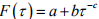• Exponential law: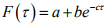f (t −τ )
• Power law: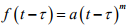• Logarithmic expression: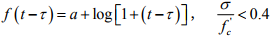where (t -τ ) is measured in days.

→ Experimental data is used to obtain coefficients.

o Prediction of creep deformation:
→ Perform short-term experimental results → Develop short-term creep curves to be used in long-term prediction.
→ When experimental data is not available, the design has to rely on a relevant code. Various code recommendations have been quite controversial.
→ ACI 209 Committee defines the creep coefficient as  ϕ (t t0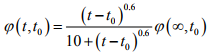where (t − t0) = time since application of load,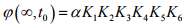= ultimate creep,

K1 and K2 relate to curing process, K3 relates to the thickness of member, K4,K5 , K6 and relate to concrete composition. (α = 2.35)

→ Principle of superposition
For analysis and design purposes the time dependent linear relation between stress and creep strain can be written in the following way: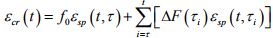where f0 = initial stress in concrete at the time τ of first loading,= additional stress increment or decrement applied at time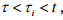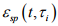= specific creep strain at time t for concrete at age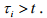→ Effect of stress on creep is predicted by the superposition method.
→ Creep strains produced in concrete at any time t by an increment of stress applied at any time τ are independent of the effects of any other stress increment applied earlier or later than time τ.
→ This method of analysis predicts creep recovery and generates stress-strain curves of a shape similar to experimental results. It is assumed that the concrete creeps in tension at the same rate as it creeps in compression.
→ Creep under variable stress can be obtained by superimposing appropriate creep curves introduced for corresponding changes in stress at different time intervals. This is true if creep is proportional to applied stress.

→ Creep under different states of stress:
o Creep takes place in tension in the same manner as in compression. The magnitude in tension is much higher.
o Creep occurs under torsional loading.
o Under unaxial compression, creep occurs not only in the axial but also in the normal directions.
o Under multiaxial loading creep occurs in all directions and affected by stresses in other normal stresses.

→ Non-linear factors in the evaluation of creep strain
o The influences of
– Temperature dependence
– Creep in combined stress
– Shrinkage o Humidity and temperature variation

→ Cross coupling of creep with shrinkage or thermal expansion.
o Cracking or strain-softening
o Cyclic loading
o High stress and multiaxial viscoplasticity

→ Examples of complex structures with significant effect of creep on deformation and failure behavior
o Thermal effect (pressure vessels, process vessels)
o Segmental concrete bridge deformation (construction and service stages)

→ Shrinkage deformation
o Shrinkage is basically a volume change of the element which is considered to occur independently of externally imposed stresses. This volume change can also be negative and is then called swelling. Thin sections are particularly susceptible to drying shrinkage and therefore must contain a certain minimum quantity of steel. In restrained concrete shrinkage results in cracking, before any loading. The minimum reinforcement is provided to control this cracking.

o Causes of shrinkage:
→ Loss of water on drying
→ Volume change on carbonation

o On average ultimate shrinkage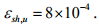o ACI 209 Committee suggests: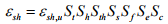where
εsh,u = ultimate factor of shrinkage,
St = factor of time,
Sh = factor of humidity,
Sth = factor of thickness,
Ss = factor of slump,
Sf = factor of fineness,
Se = factor of air content, and
Sc = factor of cement property

Offer running on EduRev: Apply code STAYHOME200 to get INR 200 off on our premium plan EduRev Infinity!

,

,

,

,

,

,

,

,

,

,

,

,

,

,

,

,

,

,

,

,

,

;